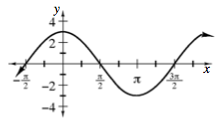### Home > INT3 > Chapter 10 > Lesson 10.2.1 > Problem10-103

10-103.

Sketch the graph of each equation below. Homework Help ✎

1. $y=3\sin\left(x+\frac{\pi}{2}\right)$

$\text{locator point} = (- \frac{\pi}{2} , 0)$

$b = 1$, period $= 2π$

amplitude $= 3$

no vertical shift, midline is $y = 0$.1. $y = −2 \sin \left(4x\right)$

$b = 4\text{, period} = \frac{2 \pi}{4} = \frac{\pi}{2}$